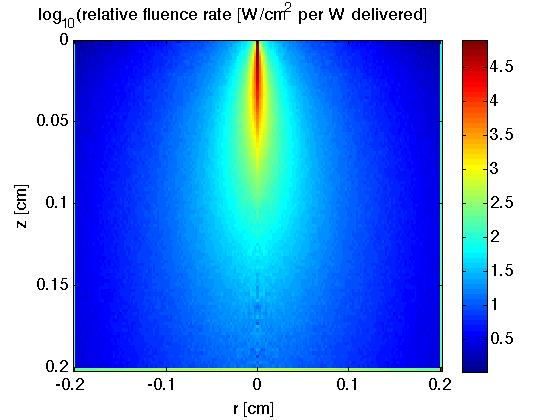A subroutine written in C language that can be called to run a Monte Carlo simulation.

An example calling program is callmcsub.c

Compile using the UNIX command:

```     cc -o gomcsub mcsubLIB.c callmcsub.c
```

Then run:

`    ./gomcsub`

which produces the output file mcOUT0.dat.

A MATLAB program lookmcsub.m reads mcOUT0.dat using a subroutine called getmcsub.m to yield the following figure:The edges of the simulation at z(NZ) and r(NR), F(NZ,:) and F(:,NR), are overflow bins, so photons absorbed at large depths >z(NZ) or radial distances >r(NR) are deposited in these overflow bins.

Other Monte Carlo programs